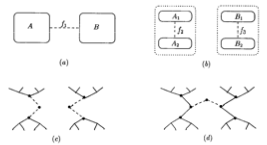## neal young / Khuller94Designing

•The traditional multi-commodity flow problem assumes a given flow network in which multiple commodities are to be maximally routed in response to given demands. This paper considers the multi-commodity flow network-design problem: given a set of multi-commodity flow demands, find a network subject to certain constraints such that the commodities can be maximally routed.

This paper focuses on the case when the network is required to be a tree. The main result is an approximation algorithm for the case when the tree is required to be of constant degree. The algorithm reduces the problem to the minimum-weight balanced-separator problem; the performance guarantee of the algorithm is within a factor of 4 of the performance guarantee of the balanced-separator procedure. If Tom Leighton and Satish Rao's balanced-separator procedure  is used, the performance guarantee is $$O(\log n)$$. This improves the $$O(\log^2 n)$$ approximation factor that is trivial to obtain by a direct application of the balanced-separator method.
Journal version of .